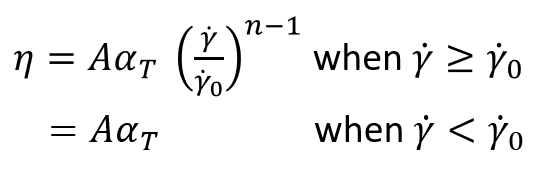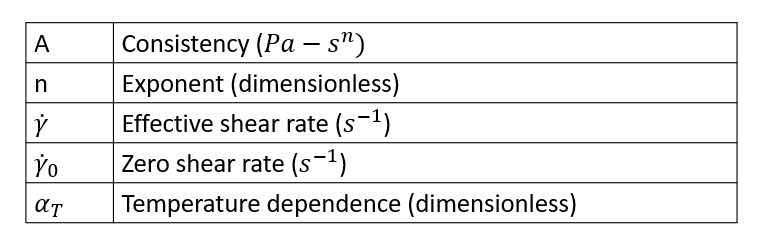# Spriggs Power Law Model

This is a three-paramter model described by the following equation.This model describes the viscosity using a power-law relationship. The exponent n determines the nature of the relationship. Unlike the power-law model where the Consistency A has complex units Pa-s^n, here the consistency is identical to viscosity. This is because the effective shear rate is normalized with zero shear rate limit. This makes the data easily understandable.

## Syntax

 Polymer PolymerName { ConstituveModel = "SpriggsPowerLaw" Density = ρ SpecificHeat = Cp (T) Conductivity = K (T) CoeffOfThermalExpansion = βT VolumetricHeatSource = Qvol Consistency = A Exponent = n ZeroShearRate = γ0 TemperatureDependence = "None" }

## Explanation of Parameters

Parameter Description Units Data Type Condition Typical Value
ConstitutiveModel Describes the model used None String Required "SpriggsPowerLaw"
Density Density fo the polymer kg/m^3 Constant Required 995.0
SpecificHeat Specific heat at constant pressure J/kg/K Constant/F(T) Required 2000.0
Conductivity Thermal conductivity W/m/K Constant/ F(T) Required 0.167
CoeffOfThermalExpansion Indicates the change in volume with change in temperature 1/K Constant Required 1.0e-05
VolumetricHeatSource Heat generated/removed in the volume by methods like electrical heating W/m^3 Constant Required 0.0
Consistency One of the parameters of the power law model. When n=1 it is the same as viscosisty. pa s^n Constant Required 1.0e+04
Exponent Power law index, defines the dependency of viscosity on shear rate. None Constant Required 0.66
ZeroShearRateLimit See above 1/s Constant Required 0.01
TemperatureDependence See Temperature Dependence None String Required "Exp(-Beta(DeltaT))"
ReferenceTemperature Temperature at which data is calculated for the intialization step. K Constant Required only if TD is not "None" 533
FreezeTemeprature This ist eh no flow temperature. Below this temperature, material ceases to flow. K Constant Required only if TD is not "none" 350
ActivationEnergy A parameter required by Arrhenius model. J/mol Constant Required only if TD is Exp(Q/RT) 16628
UniversalGasConstant A parameter from state equation PV=nRT, R is universal gas constant. J/mol/K Constant Required only if TD is Exp (Q/RT) 8.314
TemperatureSensitivity A derived parameter which has the same physical meaning as Q/R. K Constant Required only if TD is Exp(Tb/T) 2000 K
WLFConstant 1 Constant C1 of WLF model None Constant Required only if TD is WLF 17.44
WLFConstant2 Constant C2 of WLF model. This is like DeltaT, hence the value is same in K and Celsius. K Constant Required only if TD is WLF 51.6
GlassTransitionTemperature Temperature below with polymer molecules ceases to move (frozen). There are few definitions of this term K Constant Required only if TD is WLF 320
Beta Parameter in the relationship Exp(-BetaDeltaT)) None Constant Required only if TD is Exp(-Beta(DeltaT)) 0.005

F(T) - Function of Temperature. Can be specified as a TABLE1 or TCL function.

TD - TemperatureDependence